# GMAT Math : Descriptive Statistics

## Example Questions

← Previous 1 3 4 5 6 7 8 9 10 11

### Example Question #1 : Range

Consider the following set of numbers:

85, 87, 87, 82, 89

What is the range?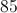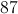Explanation:

The range is the difference between the maximum and minimum value.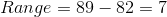### Example Question #1 : Calculating Range

What is the range for the following data set: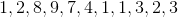Explanation:

The range is the highest value number minus the lowest value number in a sorted data set:We need to sort the data set: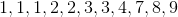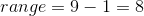### Example Question #3 : Range

What is the range for the following set: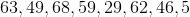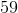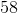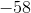Explanation:

The range is the difference between the highest and lowest number.

First sort the set: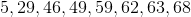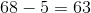### Example Question #1 : Descriptive Statistics

Below is the stem-and-leaf display of a set of test scores.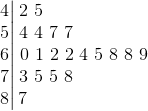What is the range of this set of scores?Explanation:

The range of a data set is the difference of the highest and lowest scores,

The numbers in the "stem" of this display represent tens digits of the test scores, and the numbers in the "leaves" represent the units digits. The highest and lowest scores represented are 87 and 42, so the range is their difference: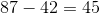.

### Example Question #1 : Descriptive Statistics

Below is the stem-and-leaf display of a set of test scores.What is the interquartile range of these test scores?Explanation:

The numbers in the "stem" of this display represent tens digits of the test scores, and the numbers in the "leaves" represent the units digits. This stem-and-leaf display represents twenty scores.

The interquartile range is the difference of the third and first quartiles.

The third quartile is the median of the upper half, or the upper ten scores. This is the arithmetic mean of the fifth- and sixth-highest scores. These scores are 73 and 69, so the mean is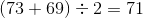The first quartile is the median of the lower half, or the lower ten scores. This is the arithmetic mean of the fifth- and sixth-lowest scores. Both of these scores are the same, however - 57.

The interquartile range is therefore the difference of these numbers: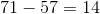### Example Question #1 : Calculating Range

Consider the data set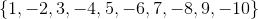.

What is its midrange?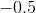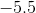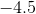Explanation:

The midrange of a data set is the arithmetic mean of its greatest element and least element. Here, those elements areand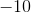, so we can find the midrange as follows: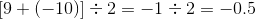### Example Question #7 : Range

Set 1: 5, 13, -2, -1, 19, 27

Set 2: 6, -3, 23, 15, m, 1

What should the value ofbe if we want the ranges of both sets of number to be equal?Explanation:

The range of a set of numbers is the difference between the highest number and the lowest number in the set.

The range of set 1 is: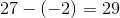The range of the second set, ignoring the value of m is: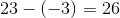We need to either subtract 3 from the lowest number in set Set 2 or add 3 to the highest number in Set 2 to get the value of m such that the range of both sets are equal.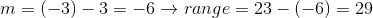or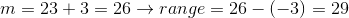### Example Question #1 : Calculating Range

Calculate the range of the following set of data: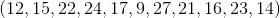Explanation:

The range of a set of data is the difference between its highest value and its lowest value, as this describes the range of values spanned by the set. A quick way to calculate the range is to locate the lowest value in the set and subtract it from the highest value, but let's arrange the set in increasing order to visualize the problem first: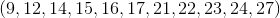Now we can see that the lowest value in the set is 9, and the highest value in the set is 27, so the range of the set is: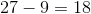### Example Question #9 : Range

Calculate the range of the following set of data: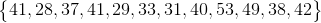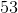Explanation:

The range of a set of data is the difference between its smallest and greatest values. We can first look through the set for the greatest value, which we can see is 53. We then look through the set for the smallest value, which we can see is 28. The range of the set is then: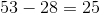### Example Question #10 : Range

Determine the mean for the following set of numbers.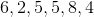Explanation:

To find the range, simply subract the smallest number from the largest. Therefore: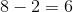← Previous 1 3 4 5 6 7 8 9 10 11

### All GMAT Math Resources